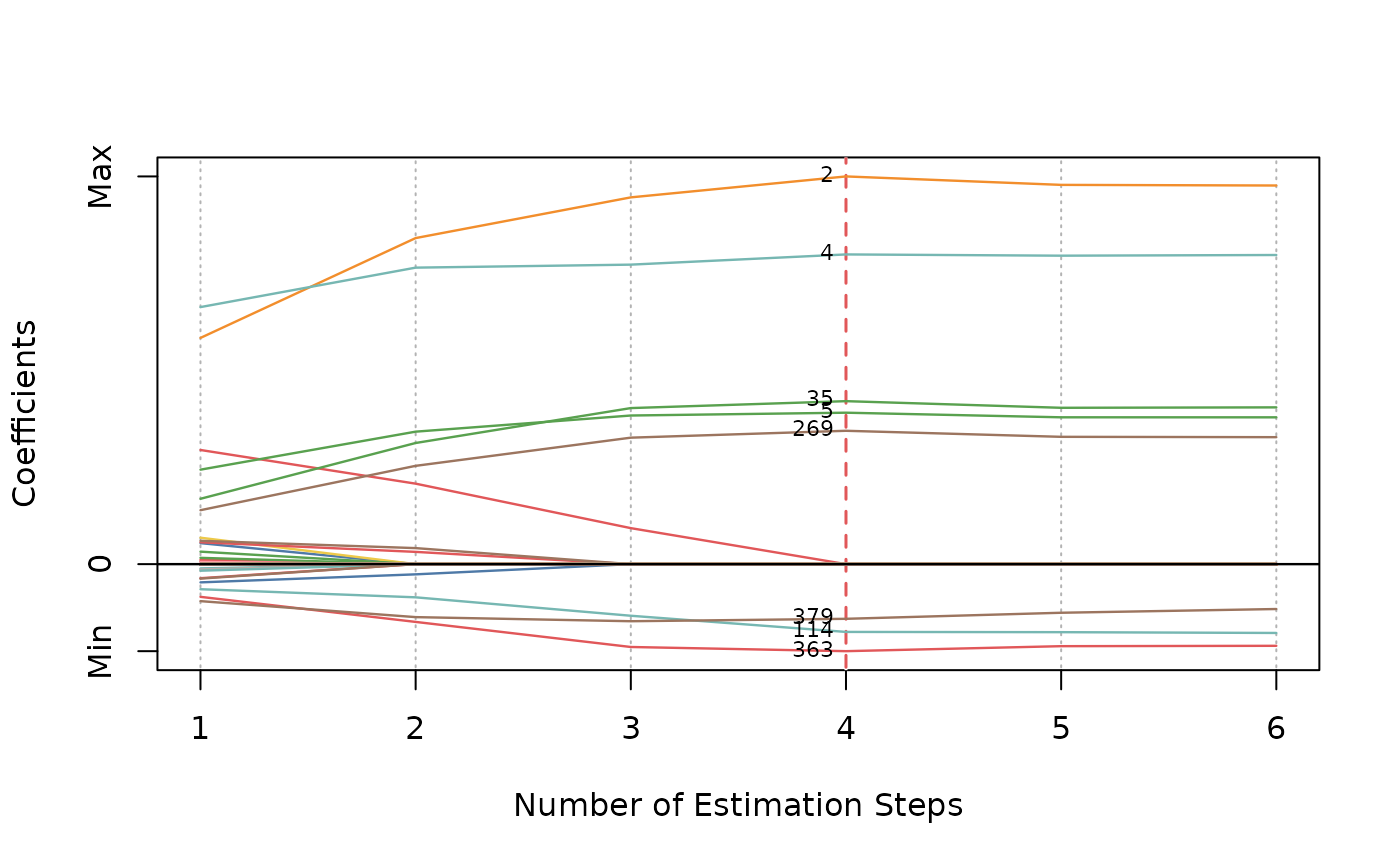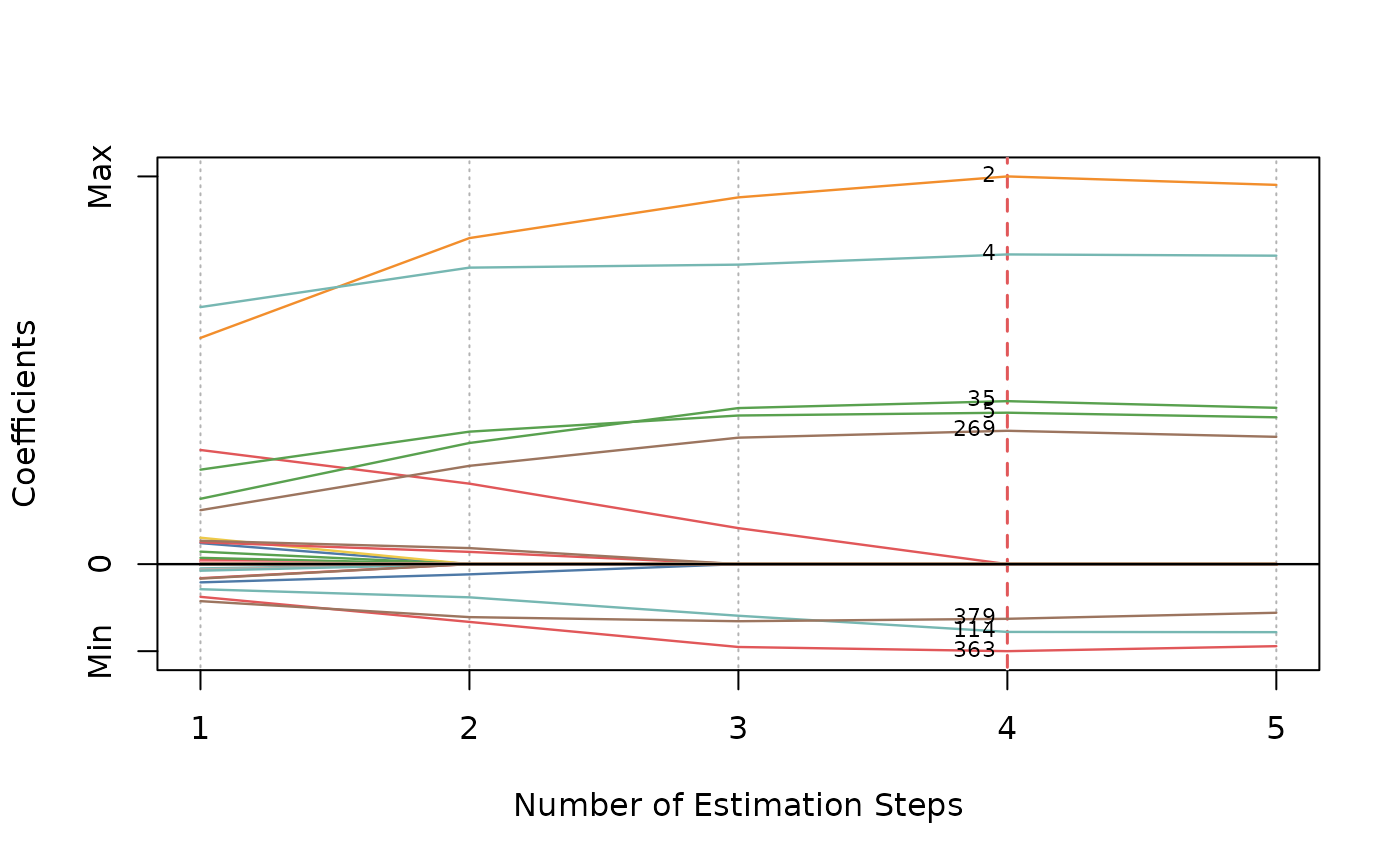Plot msaenet model objects.

## Usage

# S3 method for msaenet
plot(
x,
type = c("coef", "criterion", "dotplot"),
nsteps = NULL,
highlight = TRUE,
col = NULL,
label = FALSE,
label.vars = NULL,
label.pos = 2,
label.offset = 0.3,
label.cex = 0.7,
label.srt = 90,
xlab = NULL,
ylab = NULL,
abs = FALSE,
...
)

## Arguments

x

An object of class msaenet produced by aenet, amnet, asnet, msaenet, msamnet, or msasnet.

type

Plot type, "coef" for a coefficient path plot across all estimation steps; "criterion" for a scree plot of the model evaluation criterion used (CV error, AIC, BIC, or EBIC); "dotplot" for a Cleveland dot plot of the coefficients estimated by the model at the optimal step.

nsteps

Maximum number of estimation steps to plot. Default is to plot all steps.

highlight

Should we highlight the "optimal" step according to the criterion? Default is TRUE.

col

Color palette to use for the coefficient paths. If it is NULL, a default color palette will be assigned.

label

Should we label all the non-zero variables of the optimal step in the coefficient plot or the dot plot? Default is FALSE. If TRUE and label.vars = NULL, the index of the non-zero variables will be used as labels.

label.vars

Labels to use for all the variables if label = "TRUE".

label.pos

Position of the labels. See argument pos in text for details.

label.offset

Offset of the labels. See argument offset in text for details.

label.cex

Character expansion factor of the labels. See argument cex in text for details.

label.srt

Label rotation in degrees for the Cleveland dot plot. Default is 90. See argument srt in par for details.

xlab

Title for x axis. If is NULL, will use the default title.

ylab

Title for y axis. If is NULL, will use the default title.

abs

Should we plot the absolute values of the coefficients instead of the raw coefficients in the Cleveland dot plot? Default is FALSE.

...

Other parameters (not used).

## Author

Nan Xiao <https://nanx.me>

## Examples

dat <- msaenet.sim.gaussian(
n = 150, p = 500, rho = 0.6,
coef = rep(1, 5), snr = 2, p.train = 0.7,
seed = 1001
)

msasnet.fit <- msasnet(
dat$x.tr, dat$y.tr,
alphas = seq(0.2, 0.8, 0.2),
nsteps = 5L, tune.nsteps = "ebic",
seed = 1003
)
#> Warning: ncvreg() is intended for pathwise optimization, not for single values of lambda.
#>   1. You are strongly encouraged to fit a path and extract the solution at the lambda value of interest, rather than use ncvreg() in this way.
#>   2. In particular, if you are using the MCP or SCAD penalties, be aware that you greatly increase your risk of converging to an inferior local maximum if you do not fit an entire path.
#>   3. You may wish to look at the ncvfit() function, which is intended for non-path (i.e., single-lambda) optimization and allows the user to supply initial values.
#> Warning: ncvreg() is intended for pathwise optimization, not for single values of lambda.
#>   1. You are strongly encouraged to fit a path and extract the solution at the lambda value of interest, rather than use ncvreg() in this way.
#>   2. In particular, if you are using the MCP or SCAD penalties, be aware that you greatly increase your risk of converging to an inferior local maximum if you do not fit an entire path.
#>   3. You may wish to look at the ncvfit() function, which is intended for non-path (i.e., single-lambda) optimization and allows the user to supply initial values.
#> Warning: ncvreg() is intended for pathwise optimization, not for single values of lambda.
#>   1. You are strongly encouraged to fit a path and extract the solution at the lambda value of interest, rather than use ncvreg() in this way.
#>   2. In particular, if you are using the MCP or SCAD penalties, be aware that you greatly increase your risk of converging to an inferior local maximum if you do not fit an entire path.
#>   3. You may wish to look at the ncvfit() function, which is intended for non-path (i.e., single-lambda) optimization and allows the user to supply initial values.
#> Warning: ncvreg() is intended for pathwise optimization, not for single values of lambda.
#>   1. You are strongly encouraged to fit a path and extract the solution at the lambda value of interest, rather than use ncvreg() in this way.
#>   2. In particular, if you are using the MCP or SCAD penalties, be aware that you greatly increase your risk of converging to an inferior local maximum if you do not fit an entire path.
#>   3. You may wish to look at the ncvfit() function, which is intended for non-path (i.e., single-lambda) optimization and allows the user to supply initial values.
#> Warning: ncvreg() is intended for pathwise optimization, not for single values of lambda.
#>   1. You are strongly encouraged to fit a path and extract the solution at the lambda value of interest, rather than use ncvreg() in this way.
#>   2. In particular, if you are using the MCP or SCAD penalties, be aware that you greatly increase your risk of converging to an inferior local maximum if you do not fit an entire path.
#>   3. You may wish to look at the ncvfit() function, which is intended for non-path (i.e., single-lambda) optimization and allows the user to supply initial values.
#> Warning: ncvreg() is intended for pathwise optimization, not for single values of lambda.
#>   1. You are strongly encouraged to fit a path and extract the solution at the lambda value of interest, rather than use ncvreg() in this way.
#>   2. In particular, if you are using the MCP or SCAD penalties, be aware that you greatly increase your risk of converging to an inferior local maximum if you do not fit an entire path.
#>   3. You may wish to look at the ncvfit() function, which is intended for non-path (i.e., single-lambda) optimization and allows the user to supply initial values.

plot(msasnet.fit)
plot(msasnet.fit, label = TRUE)plot(msasnet.fit, label = TRUE, nsteps = 5)plot(msasnet.fit, type = "criterion")plot(msasnet.fit, type = "criterion", nsteps = 5)plot(msasnet.fit, type = "dotplot", label = TRUE)
plot(msasnet.fit, type = "dotplot", label = TRUE, abs = TRUE)